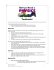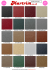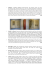# CSE 101 Homework 4 - UCSD VLSI CAD Laboratory

## Transcription

CSE 101 Homework 4 - UCSD VLSI CAD Laboratory
```CSE 101 Homework 4
February 20, 2015
CSE 101: Design and Analysis of Algorithms
Winter 2015
Homework 4
Due: February 26, 2015
1. Suppose that you are given a set of n jobs, and that each job has a starting time, an ending time that
is strictly greater than the starting time, and a profit associated with completing the job (so if a job
is not completed then you get no profit). If scheduled, jobs must run from their starting time to their
ending time. Find an efficient algorithm that takes in the set of jobs and returns the set of jobs such
that no two jobs overlap and the total profit for completing the jobs is maximized. You can assume for
simplicity that that starting and ending times are integers, and that if a job ends at time t the next
job cannot start until time t + 1.
2. Given a string, find an efficient algorithm that computes the minimum number of letters that must be
inserted to make the string into a palindrome. For example, it would take two insertions to turn the
word ”loyal” into ”loayaol” and four insertions to turn ”aaaabbb” into ”aaaabbbaaaa”.
3. (DPV textbook, Problem 6.14.) Cutting cloth. You are given a rectangular piece of cloth with dimensions X × Y , where X and Y are positive integers, and a list of n products that can be made using the
cloth. For each product i ∈ [1, n] you know that a rectangle of cloth of dimensions ai × bi is needed
and that the final selling price is ci . Assume the ai , bi , and ci are all positive integers. You have a
machine that can cut any rectangular piece of cloth into two pieces either horizontally or vertically.
Design an algorithm that determines the best return on the X × Y piece of cloth, that is, a strategy
for cutting the cloth so that the products made from the resulting pieces give the maximum sum of
selling prices. You are free to make as many copies of a given product as you wish, or none if desired.
4. (DPV textbook, Problem 6.22.) Give an O(nt) algorithm for the following task.
Input: A list of n positive integers a1 , a2 , ..., an ; a positive integer t
Question: Does some subset of the ai s add up to t? (You can use each ai at most once.)
5. (DPV textbook, Problem 7.21.) An edge of a flow network is called critical if decreasing the capacity
of this edge results in a decrease in the maximum flow. Give an efficient algorithm that finds a critical
edge in a network.
6. (DPV textbook, Problem 7.23.) The vertex cover of an undirected graph G = (V, E) is a subset of
the vertices which touches every edge-that is, a subset S ⊂ V such that for each edge u, v ∈ E, one or
both of of u, v are in S.
Show that the problem of finding the minimum vertex cover in a bipartite graph reduces to maximum
flow. (Hint : can you relate this to the minimum flow in a related network?)
7. (DPV textbook, Problem 7.11.) Write the dual to the following linear program.
max x + y
2x + y ≤ 3
x + 3y ≤ 5
x, y ≥ 0
Find the optimal solutions to both primal and dual linear programs.
1
```

### CSE 101 Homework 3 - UCSD VLSI CAD Laboratory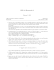### Glass instructions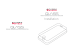### Guest Book Options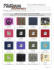### Shop Online: JamiMark.norwex.biz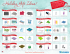### Maintenance and care instructions REV A.cdr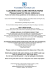### 10020 Pendleton - Standard Furniture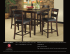### Engineering 9 – Statics Sample Test for Exam #2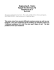### Let Us Get You There!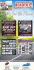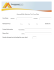### Document 6512841